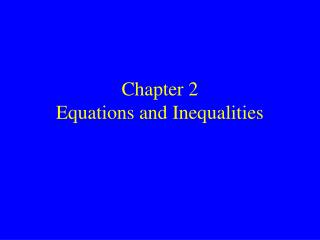DownloadDownload PresentationChapter 2 Equations and Inequalities

# Chapter 2 Equations and Inequalities

Télécharger la présentation## Chapter 2 Equations and Inequalities

- - - - - - - - - - - - - - - - - - - - - - - - - - - E N D - - - - - - - - - - - - - - - - - - - - - - - - - - -
##### Presentation Transcript

1. Chapter 2Equations and Inequalities

2. No HW Quiz #1.9-10Pass Your HW to the front!

3. 2.1 More on Solving Equations

4. Objective: Solve equations containing fractions or decimals. When an equation contains fractions or decimals, we can use the multiplication property to eliminate them. The process is called clearing the equation offractions or decimals.

5. Solving an Equation with Decimals Solve the equation by clearing it of all decimals first.

6. A B C Solve the equation by clearing it of all fractions or decimals first.

7. Objective: Use the principle of zero products to solve equations. Solving an Equation with Zero Products

8. Solving an Equation with Zero Products

9. Solving an Equation with Zero Products Solve the equation using the zero products property.

10. Solving an Equation Solve the equation

11. Solving an Equation Solve the equation

12. 2.2 Using Equations

13. Objective: Solve problems by translating to equations.

14. Objective: Solve problems by translating to equations. A 23-ft cable is cut into two pieces, one three times as long as the other. How long are the pieces?

15. Herman is doing an experiment that calls for a 40% solution of copper sulfate. He has a 60ml of a solution that is 25% copper sulfate. How many milliliters of a 70% solution should Herman add to obtain the required 40% solution?

16. Two cars leave the 7-11 at the same time going in the same direction. One car is going 45 mph and the other car is going 58 mph. In 2.5 hours how far apart are they?

17. A popular music CD was discounted 25% to \$8.42. What was the original price?

18. Write an equation and use it to solve the following F A tank at a marine exhibit contains 2000 gallons of sea water. The sea water is 7.5% salt. How many gallons, to the nearest gallon, of fresh water must be added to the tank so that the mixture contains only 7% salt?

19. HW #2.1-2Pg 64 15-41 Odd, 42-46Pg 70 19-27 Odd

20. HW Quiz #2.1-2Tuesday, March 11, 2014

21. 2.3 Solving Formulas

22. Objective: Solve a formula for a specified letter. Solving a Formula for a specific variable A formula is a rule for doing a specific calculation.

23. Solving a Formula for a specific variable

24. Solving a Formula for a specific variable

25. Solving a Formula for a specific variable

26. 2.4 Solving Inequalities2.5 Using Inequalities

27. Objective: Determine if a number is a solution of an inequality and graph the solution set. If a number occurs to the left of another on the number line, the first number is less than the second, and the second is greater than the first.

28. Objective: Determine if a number is a solution of an inequality and graph the solution set. Determine whether the given number is a solution of the inequality. x + 3 < 6;5 3 - x < 2; 4 3x + 2  4x - 3; 5

29. Determine whether the given number is a solution of the inequality. C: x - 3 < 6;5 D: 3x + 2  4x - 3; 6

30. Solving an Inequality

31. Objective: Solve and graph inequalities using the multiplication property.

32. Solving an Inequality

33. Solving an Inequality

34. F E

35. Solving an Inequality

36. Solving an Inequality

37. HW Quiz #2.3-5Tuesday, March 11, 2014

38. 2.6 Compound Inequalities

39. Objective: Solve compound inequalities. A compound inequality is two simple inequalities joined by “and” or “or.” Statements involving the word “or” are called disjunctions. A disjunction is true when at least one of the statements is true Statements involving the word “and” are called conjunctions. For a conjunction to be true all individual statements must be true

40. Conjunctions In mathematics, conjunction is called intersection and is symbolized by the symbol: 

41. Disjunctions In mathematics, disjunction is called union and is symbolized by the symbol: 

42. Solve the following inequalities. Graph your solution on a number line and write them in interval notation.

43. Write and solve a compound inequality that is the conjunction of two disjunctions. Write an inequality that has no solution and show it has no solution. Write an inequality whose solutions are all real numbers and show why the solutions are all real numbers.